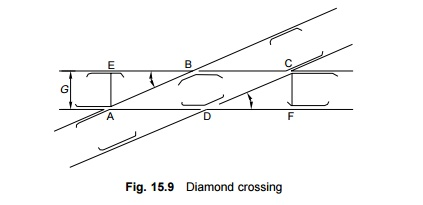Home | | Railways and Airports and Harbour Engineering | Railway Track Junctions: Diamond Crossing

# Railway Track Junctions: Diamond CrossingA diamond crossing is provided when two tracks of either the same gauge or of different gauges cross each other. It consists of two acute crossings (A and C) and two obtuse crossings (B and D).

Diamond Crossing

A diamond crossing is provided when two tracks of either the same gauge or of different gauges cross each other. It consists of two acute crossings (A and C) and two obtuse crossings (B and D). A typical diamond crossing consisting of two tracks of the same gauge crossing each other, is shown in the Fig. 15.9.

In the layout, ABCD is a rhombus with four equal sides. The length of the various constituents may be calculated as follows.

EB = DF = AE cot a = GN

AB = BC = G coseca

Diagonal AC = G coseca/2

Diagonal BD = G seca/2It can be seen from the layout that the length of the gap at points B and D increases as the angle of crossing decreases. Longer gaps increase the chances of the wheels, particularly of a small diameter, being deflected to the wrong side of the nose. On Indian Railways, the flattest diamond crossing permitted for BG and MG routes is 1 in 8.5.

Along with diamond crossings, single or double slips may also be provided to allow the vehicles to pass from one track to another.

1 Single Slip and Double Slip

In a diamond crossing, the tracks cross each other, but the trains from either track cannot change track. Slips are provided to allow vehicles to change track.

The slip arrangement can be either single slip or double slip. In single slips, there are two sets of joints, the vehicle from only one direction can change tracks. In the single slip shown in Fig. 15.10, the train on track A can change to track D, whereas the train on track C remains on the same track, continuing onto track D.In the case of double slips, there are four sets of points, and trains from both directions can change tracks. In the double slip shown in Fig. 15.11, the trains on both tracks A and C can move onto either track B or D.2 Improvements in the Design of Diamond Crossings

In order to smoothen the ride over a diamond crossing, the following improvements are generally made.

(a)  Provision of 25 mm higher check rails

(b) Reduction in the check rail clearance by 3 mm in the case of obtuse crossings.

Example 15.4 Two BG tracks cross each other at an angle of 1 in 10. Calculate the important dimensions of the diamond crossing.

Solution      The data given are as follows (Fig. 15.9):

(i)  Number of the crossing (N) = 10 Gauge (G) = 1.676 m

(ii)   N = cot a or 10 = cot a

Therefore, a = 5 o 42 38��

(iii)   EB = DF = AE cota = GN

= 1.676 � 10 = 16.76 m

(iv)  AB = BC = G coseca

=1.676 �  10.05 = 16.85 m

(v) AC = G coseca/2

=       1.676 � 20.10 = 33.70 m

(vi)                       BD = G sec a/2

= 1.70 m

Study Material, Lecturing Notes, Assignment, Reference, Wiki description explanation, brief detail
Civil : Railway Airport Harbour Engineering : Railway Engineering : Track Junctions and Simple Track Layouts : Railway Track Junctions: Diamond Crossing |